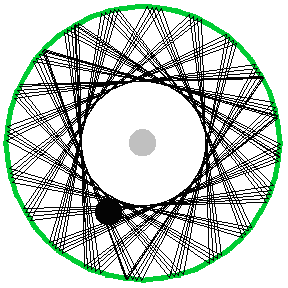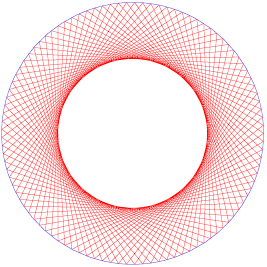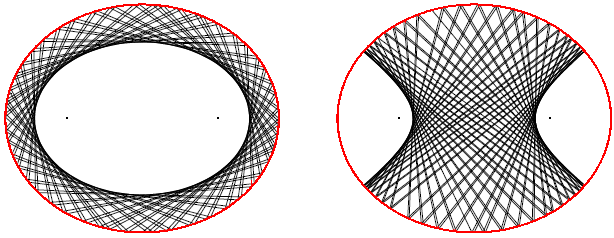MATH EXPLORERS' CLUB Cornell Department of Mathematics

# Billiards and Puzzles

 Introduction1. Rectangular Billiards 2. Circular/Elliptical Tables 3. Triangular Billiards 4. Puzzles 5. Two-Hole Billiards References## Circular Tables

Let us now assume that the billiard table is a circle rather than a polygon. The rules remain the same in principle, but we will summarize them below. Consider that a red ball hits the circle from the inside at a point P Then take the tangent to the circle at that point. The incoming angle, say a, that the trajectory makes with the tangent is equal to the outgoing angle, say b, of the same lines, as shown in the figure. The situation is the same if we consider an ellipse instead of the circle: the ball bounces with respect to the tangent, as in the case of the rectangular billiards table.Example: Consider the following amusing puzzle from MatheMUSEments, Reference #5. Suppose there is a pocket in the center of a circular table, depicted grey, and a black ball. We wish to put the black ball into the pocket. Assume that there is no friction and so a ball could bounce forever. It turns out that this is not always possible. The black ball can follow a path that never passes close to the center of the table, therefore never hitting the pocket.Consider again the question from, Reference #7, but not on a rectangular billiard table, but on a circular one.

Example: We start with a ball on the circle and we ast whether it is possible to hit it in such a way so that it returns to the original position after exactly 6 bounces. The answer is positive and we call such trajectories periodic.However, there are also nonperiodic trajectories. In other words, it is possible to shoot a ball located on the margin in such a way so that the trajectory never returns back at the same spot. In fact the trajectory will not enter at all in a small disc centered at the center of the table, but will fill completely the remaining of the table. A multi-bounces trajectory is depicted below. In order to obtain such a picture one has to shoot the ball situated on the circle at an incidence angle (say a, as in the first picture) that is irrational multiple of π.Remark: It is not possible for the trajectory of a moving ball to cover the whole disc completely, i.e. to be dense in the entire disc.

## Elliptical Tables

Let us look at an elliptical billiard table, where there are even more surprising and unusual features, also described in Reference #4, Reference #2,. Suppose that we have a pocket at one focus of the ellipse and a ball at the other focus, and that we wish to put the ball in its pocket. We shoot in any direction, hit the cushion, bounce off and enter the pocket immediately. In this case one does not need any accuracy to hit the pocket.If we now have only one ball placed at a focus and shoot in any direction, then the ball bounces from the cushion, passes over the other focus and continues to pass over a focus. Not many bounces are needed to notice that the trajectory of the ball will eventually follow the major axis of the ellipse.Suppose now that the ball does not start at a focus. If the trajectory of the ball does not pass between the foci, then the ball avoids a smaller ellipse (with the same foci) in the middle. The trajectory is then dense in the region between the two ellipses. If however the trajectory passes between the two foci, then the trajectory describes a hyperbola with the same foci as the elliptical table. We assume of course that the ball bounces from the cushion forever, i.e. there is no friction. Thus the ball does not enter at all in a region described by a hyperbola with the same foci. The pictures below describe these phenomena:Top Next lesson